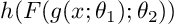# How to optimize two sets of parameters and jump out local minima?

I have a function that has a structure like this:x are input, theta_1 and theta_2 are two sets of trainable parameters. g and h are two defined differentiable functions. h outputs a scalar. F is a fully connected neural network. The number of parameters theta_1 is much smaller than the number of parameters theta_2. (say theta_1 represents 10 trainable parameters and theta_2 represents 1000 trainable parameters) My goal is to minimize this function and get the corresponding value of theta_1.

I initialize theta_1 based on prior information and initialize theta_2 randomly. From my experiments, the function stays in local minima if I have a bad initial of theta_1. I tried to use `torch.optim.lr_scheduler.` `CosineAnnealingLR` and a large learning rate to jump out of the local minimum. But it doesn’t always work.
I wonder whether there are any methods that can help me to avoid local minimal. Like learning rate scheduler strategy or design different structure of F.

Thanks!